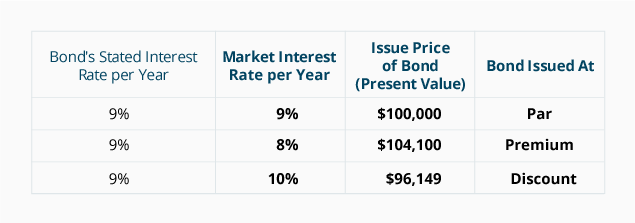# Amortizing Bond Discount with the Effective Interest Rate Method

When a bond is sold at a discount, the amount of the bond discount must be amortized to interest expense over the life of the bond. Since the debit amount in the account Discount on Bonds Payable will be moved to the account Interest Expense, the amortization will cause each period's interest expense to be greater than the amount of interest paid during each of the years that the bond is outstanding.

The preferred method for amortizing the bond discount is the effective interest rate method or the effective interest method. Under the effective interest rate method the amount of interest expense in a given accounting period will correlate with the amount of a bond's book value at the beginning of the accounting period. This means that as a bond's book value increases, the amount of interest expense will increase.

Before we demonstrate the effective interest rate method for a 5-year 9% \$100,000 bond issued in a 10% market for \$96,149, let's highlight a few points:

1. The bond discount of \$3,851 must be amortized to Interest Expense over the life of the bond. The amortization will cause the bond's book value to increase from \$96,149 on January 1, 2022 to \$100,000 just prior to the bond maturing on December 31, 2026.

2. The corporation must make an interest payment of \$4,500 (\$100,000 x 9% x 6/12) on each June 30 and December 31 that the bonds are outstanding. The Cash account will be credited for \$4,500 on each of these dates.

3. The effective interest rate is the market interest rate on the date that the bonds were issued. In our example the market interest rate on January 1, 2022 was 5% per semiannual period for 10 semiannual periods.

4. The effective interest rate is multiplied times the bond's book value at the start of the accounting period to arrive at each period's interest expense.

5. The difference between Item 2 and Item 4 is the amount of amortization.

The following table illustrates the effective interest rate method of amortizing the \$3,851 discount on bonds payable: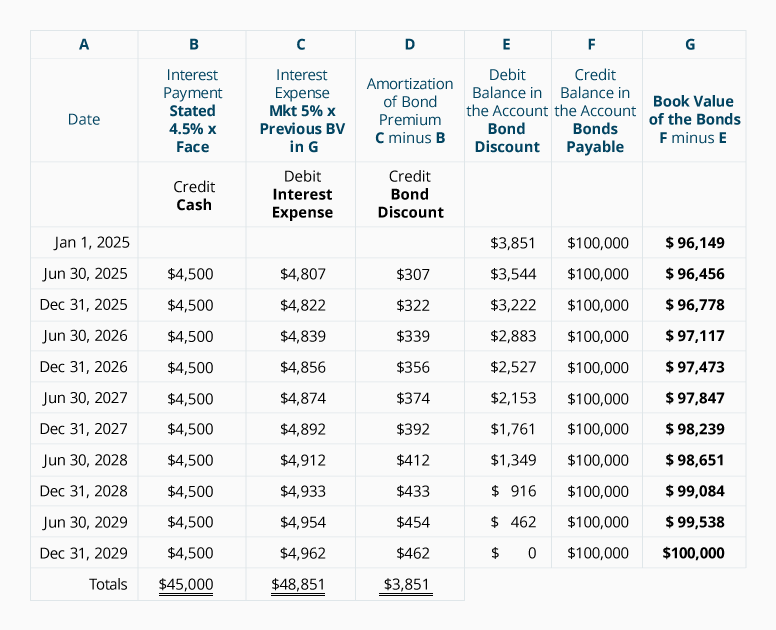Let's make a few points about the above table:

• Column B shows the interest payments required by the bond contract: The bond's stated rate of 9% per year divided by two semiannual periods = 4.5% per semiannual period multiplied times the face amount of the bond.

• Column C shows the interest expense. This calculation uses the market interest rate at the time the bonds were issued: The market rate of 10% per year divided by two semiannual periods = 5% semiannually.

• The interest expense in column C is the product of the 5% market interest rate per semiannual period times the book value of the bond at the start of the semiannual period. Notice how the interest expense is increasing with the increase in the book value in column G. This correlation between the interest expense and the bond's book value makes the effective interest rate method the preferred method for amortizing the discount on bonds payable.

• Because the present value factors that we used were rounded to three decimal positions, our calculations are not as precise as the amounts determined by use of computer software, a financial calculator, or factors that were carried out to more decimal places. As a result, our amortization amount in 2026 required a slight adjustment.

If the company issues only annual financial statements and its accounting year ends on December 31, the amortization of the bond discount can be recorded on the interest payment dates by using the amounts from the schedule above. In our example, there is no accrued interest at the issue date of the bonds and at the end of each accounting year because the bonds pay interest on June 30 and December 31. The entries for 2022, including the entry to record the bond issuance, are shown next.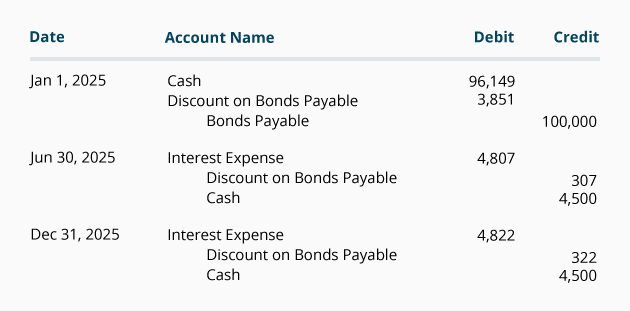The journal entries for the year 2023 are: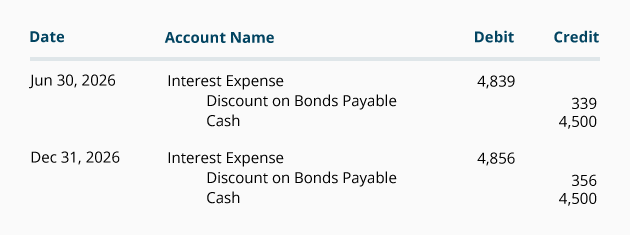The journal entries for the years 2024 through 2026 will also be taken from the schedule shown above.

### Comparison of Amortization Methods

Below is a comparison of the amount of interest expense reported under the effective interest rate method and the straight-line method. Note that under the effective interest rate method the interest expense for each year is increasing as the book value of the bond increases. Under the straight-line method the interest expense remains at a constant amount even though the book value of the bond is increasing. The accounting profession prefers the effective interest rate method, but allows the straight-line method when the amount of bond discount is not significant.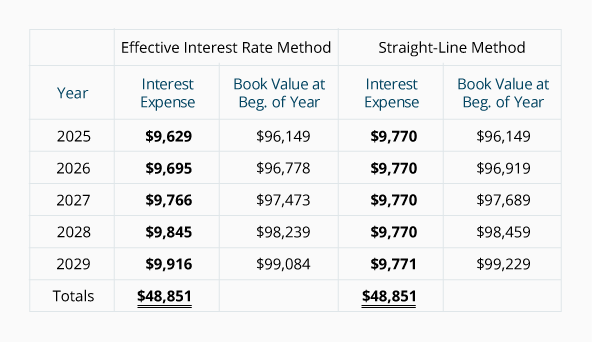Notice that under both methods of amortization, the book value at the time the bonds were issued (\$96,149) moves toward the bond's maturity value of \$100,000. The reason is that the bond discount of \$3,851 is being reduced to \$0 as the bond discount is amortized to interest expense.

Also notice that under both methods the total interest expense over the life of the bonds is \$48,851 (\$45,000 of interest payments plus the \$3,851 of bond discount.)

### Summary of the Effect of Market Interest Rates on a Bond's Issue Price

The following table summarizes the effect of the change in the market interest rate on an existing \$100,000 bond with a stated interest rate of 9% and maturing in 5 years.